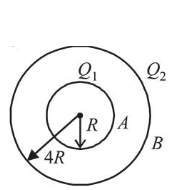# Concentric metallic hollow spheres of radii R and 4 R hold charges

Question:

Concentric metallic hollow spheres of radii $R$ and $4 R$ hold charges $Q_{1}$ and $Q_{2}$ respectively. Given that surface charge densities of the concentric spheres are equal, the potential difference $V(R)-V(4 R)$ is :

1. (1) $\frac{3 Q_{1}}{16 \pi \varepsilon_{0} R}$

2. (2) $\frac{3 Q_{2}}{4 \pi \varepsilon_{0} R}$

3. (3) $\frac{Q_{2}}{4 \pi \varepsilon_{0} R}$

4. (4) $\frac{3 Q_{1}}{4 \pi \varepsilon_{0} R}$

Correct Option: 1

Solution:

(1) We have given two metallic hollow spheres of radii $R$ and $4 R$ having charges $Q_{1}$ and $Q_{2}$ respectively.

Potential on the surface of inner sphere (at $A$ )

$V_{A}=\frac{k Q_{1}}{R}+\frac{k Q_{2}}{4 R}$

Potential on the surface of outer sphere (at $B$ )

$V_{B}=\frac{k Q_{1}}{4 R}+\frac{k Q_{2}}{4 R}$         $\left(\right.$ Here, $\left.k=\frac{1}{4 \pi \varepsilon_{0}}\right)$Potential difference,

$\Delta V=V_{A}-V_{B}=\frac{3}{4} \cdot \frac{k Q_{1}}{R}=\frac{3}{16 \pi \in_{0}} \cdot \frac{Q_{1}}{R}$